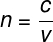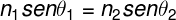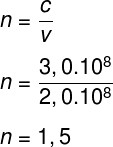Optics

# refraction of light

Refraction of light is a phenomenon that occurs in electromagnetic waves that are transmitted through some translucent or transparent medium. When light penetrates refractive media, that is, those capable of refracting light, its speed decreases. Furthermore, according to the angle of incidence, it is possible for light to be deflected so that its direction of propagation undergoes changes.

The refraction of light is determined by the decrease in its propagation speed . Such a decrease, in turn, is measured by means of a number called the refractive index . The refractive index measures the ratio of the speed of light in a vacuum to the speed of light in other media.

## Refraction and speed of light

In a vacuum, the speed of light has its limit value: approximately 300 thousand kilometers per second (c = 3.0 x 10 5 km/s). When light passes through some transparent medium, such as air or water, its speed is slowed down to a fraction of its speed in vacuum . The more refractive an optical medium, the greater the reduction in the speed of light. In diamond, for example, whose refractive index is 2.4, the speed of light is about 2.4 times slower than in a vacuum.

In addition to the change in speed, light can also undergo variations in its angle of incidence . This occurs when it crosses the interface between two media of different refractive indices. However, this lateral deviation does not occur if the light falls perpendicularly to the surface that separates two optical media. Want to know more about how this deflection occurs? Access our text on Snell’s law .

In the phenomenon of refraction, the frequency of light does not change, however, its speed and wavelength vary inversely proportionally: if the speed of light decreases, its wavelength increases and vice versa.

## Refractive index

The refractive index is calculated by dividing the speed of light in a vacuum by the speed of light in a given optical medium. Furthermore, the refractive index of a medium also varies according to the frequency of incident light , for example: the refractive index for the frequency of violet light is greater than the index of refraction for red light.

It is for this reason that light can be scattered after being transmitted through a prism , giving rise to the visible electromagnetic spectrum , as occurs in rainbows. Later, we will see how we can calculate the refractive index.

## Light refraction formulas

The refraction of light can be calculated using a few simple formulas. The following formula allows you to calculate the refractive index of some medium, check it out:n – refractive index

c – speed of light in vacuum (c = 3.0 x 10 8 m/s)

v – speed of light in other media

Another important formula for the refraction of light is known as the Snell-Descartes law. This allows us to calculate the angle of refraction of light, for example. Check out:1 en 2 – refractive index of media 1 and 2

θ 1 and θ 2 – angles of incidence and refraction, respectively

Using the two formulas, it is possible to solve most of the exercises and situations that involve the refraction of light.

## Exercises on the refraction of light

Question 1) Refraction of light occurs when light passes through some refracting medium. Regarding this process, we can say that:

a) in the process of refraction, the frequency of light does not change.

b) in the process of refraction, the speed of light remains constant.

c) when undergoing refraction, the speed of light and its wavelength decrease.

d) in refraction, the speed of light can only decrease.

e) in refraction, the speed of light can only increase.

Template: Letter A

Resolution:

During the process of refraction of light, its speed changes as well as its wavelength, however, its frequency remains constant. Therefore, the correct alternative is letter A.

Question 2) When passing through a glass, the speed of a ray of light becomes 2.0 x 10 8 m/s. The refractive index of this medium is:

Data: c = 3.0.10 8 m/s

a) 3.0

b) 2.0

c) 1.5

d) 0.5

e) 1.25

Template: letter B

Let’s calculate the refractive index of the medium, note:Resolution:

According to the calculation made, the refractive index of this glass is 1.5, so the correct alternative is the letter C.

Question 3) The phenomenon responsible for the optical illusion that gives rise to the mirages is known as

a) reflection.

b) refraction.

c) diffraction.

d) dispersion.

e) scattering.

Template: letter B

Resolution :

Mirages arise when atmospheric gas undergoes great heating. When this occurs, the refractive index of the air undergoes changes, producing the visual effects that give rise to mirages.

Question 4) The refractive index of a transparent optical medium is 1.8. In this regard, it is possible to state that

a) The speed of light in this medium is 1.8 times faster than in a vacuum.

b) the speed of light in this medium is 1.8 times slower than in a vacuum.

c) the frequency of refracted light in this medium is 1.8 times lower than in a vacuum.

d) the wavelength does not change.

e) the wavelength increases.

Template: letter B

Resolution:

As we know, the refractive index measures the ratio between the speed of light in media and the speed of light in a vacuum. Therefore, a refractive index of 1.8 indicates that light moves 1.8 times faster in a vacuum than in a medium with this refractive index. Furthermore, when it undergoes refraction, light does not change its frequency, and in this case, its wavelength decreases by 1.8 times. Therefore, the correct alternative is letter B.